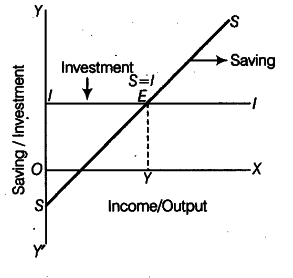# When is an economy in equilibrium?

When is an economy in equilibrium ? Explain with the help of saving and investment functions. Also explain the changes that take place in an economy when the economy is not in equilibrium. Use diagram.

Equilibrium level of income is determined at a point where ex-ante or planned saving is equal to planned investment. This is because, in equilibrium:
AS = AD or C + S= C + I or S = I
E is the point where S= I, hence, the point at which the economy is in equilibrium. OY is the equilibrium level of National Income.
What happens when S > I?
When savings are greater than investment in an economy, it refers to AD < AS. There will be a rise in inventory stock and prices will start to fall. To clear their stocks, the producers will now plan lesser output.
This will mean lesser income in the economy. Lesser income implies lesser saving. The process will continue till S = I.
What Happens if S < I? In case S< I, it implies a situation when withdrawal of expenditure (S) is lesser than • injection of expenditure (7 ) into the circular flow of income. Accordingly, overall expenditure in the economy would exceed than what is required to buy the planned output. It is a situation of higher AD than AS.
To cope with the situation, the producers would now plan higher output. Higher output would mean higher income and higher saving. The process will continue till S = I.